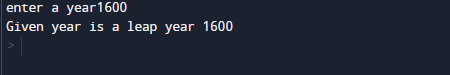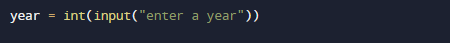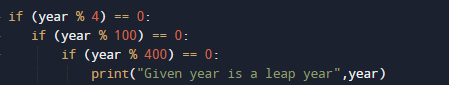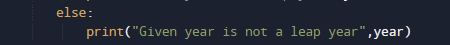# Python Program to Check Leap Year

In this tutorial you will learn about the Python Program to Check Leap Year and its application with practical example.

In this tutorial, we will learn to create a Python Program to Check enter year is a Leap Year  or not using Python programming.

## Prerequisites

Before starting with this tutorial we assume that you are best aware of the following Python programming topics:

• Python Operator.
• Basic Input and Output
• Basic Python  programming.
• Python if-else statements.
• Python Loop.

## What is Leap Year?

Leap Year:
If the value given by user is completely divisible by 4, 100 and 400 then it is a leap year.
If the given value by user is divisible by 4 but not  by 100 then it is a leap year.

Not a Leap Year:
If the value  given by user  is not divisible by 4 then it is not a leap year
If the given value is divisible by 4 and 100 but not divisible by 400 then it is not a leap year.

For example,

• 2000 is a leap year.
• 1890 is not  a leap year.

## Python Program to Check Leap Year

In our program below we will check condition for Leap year.First we would declared and initialized the required variables.Next, we would prompt user to input the value for Year. Later we will find entered year by user is leap year or not.

## Output

Leap yearNot a Leap year.In our program, we have first declared and initialized a set variables required in the program.

• year =taking year value from user.

First of all  we declare variable and take a value from user to check whether a year is leap or not is.After taking values from user we will check three different conditions on a given year

1. If given year is completely divisible by 4 (year % 4==0).
2. And complete  divisible by 100 i.e ( year % 100==0)
3. Also Divisible by  400 ( year % 400 == 0 ).

If all three conditions satisfied then the Year is a Leap Year.A year is leap year if it is exactly divisible by 4 except for century years (years ending with 00). Year is leap year if century year perfectly divisible by 400.

if all these condition falls then the entered year is a not a leap year.In this tutorial we have learn about the Python Program to Check Leap Year and its application with practical example. I hope you will like this tutorial.